# Perimeter + Pythagorean theorem - math problems

#### Number of problems found: 119

• Find the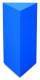Find the weight of a wooden regular triangular prism with a height equal to the perimeter of the base and a figure inscribed in a circle with a radius of 6, M cm, where M is the month of your birth. The density of oak is 680 kg/m ^ 3.
• Trapezoid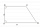The rectangular trapezoid ABCD with right angle at the vertex A has sides a, b, c, d. Calculate the circumference and the area of the trapezoid if given: a = 25cm, c = 10cm, d = 8cm
• Diagonals of rhombus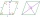Find a length of the diagonal AC of the rhombus ABCD if its perimeter P = 112 dm and the second diagonal BD has a length of 36 dm.
• Centre of the hypotenuseFor the interior angles of the triangle ABC, alpha beta and gamma are in a ratio of 1: 2: 3. The longest side of the AB triangle is 30 cm long. Calculate the perimeter of the triangle CBS if S is the center of the side AB.
• Triangular landJana has a rectangular garden measuring 30 meters by 72 meters that she wants to split diagonally from corner to corner using a fence. How long does her fence need to be?
• Angle of diagonalsCalculate the perimeter and the area of a rectangle if its diagonal is 14 cm and the diagonals form an angle of 130°.
• Side and diagonalFind the circumference and the area of the rectangle if given: side a = 8 cm diagonal u = 10 cm.
• Pentagonal pyramidThe height of a regular pentagonal pyramid is as long as the edge of the base, 20 cm. Calculate the volume and surface area of the pyramid.
• Ratio in trapeziumThe height v and the base a, c in the trapezoid ABCD are in the ratio 1: 6: 3, its content S = 324 square cm. Peak angle B = 35 degrees. Determine the perimeter of the trapezoid
• Garden GThe rectangular, trapezoidal garden has a base length of 81m, 76m, and a vertical arm of 12m. Calculate how many m2 of the area will remain for planting greenery if 1/3 of the area is built. Calculate the consumption of mesh for land fencing.
• Determine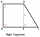Determine which type of quadrilateral ABCD is and find its perimeter if you know coordinates of vertices: A/2,4 /, B / -2,1 /, C / -2, -2 /, D/2, -5 /.
• Maximum of volumeThe shell of the cone is formed by winding a circular section with a radius of 1. For what central angle of a given circular section will the volume of the resulting cone be maximum?
• Surface and volumeFind the surface and volume of the rotating cone if the circumference of its base is 62.8 m and the side is 25 m long.
• Trip with compassDuring the trip, Peter went 5 km straight north from the cottage, then 12 km west and finally returned straight to the cottage. How many kilometers did Peter cover during the whole trip?
• Difference of legsIn a right triangle, the length of the hypotenuse is 65 m, and the difference of legs is 23 m. Calculate the perimeter of this triangle.
• Base of an isosceles triangleCalculate the size of the base of an isosceles triangle, the height is 5 cm and the length of the arm is 6.5 cm. What is the perimeter of this triangle?
• Medians in RT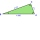The rectangular triangle ABC has a length of 10 cm and 24 cm. Points P, Q, R are the centers of the sides of this triangle. The perimeter of the PQR triangle is:
• Triangular prism - regularThe regular triangular prism is 7 cm high. Its base is an equilateral triangle whose height is 3 cm. Calculate the surface and volume of this prism.
• Horses playgroundThe fence for the horses has the shape of a rectangular trapezoid with an area of 400 m2, the base lengths should be 31 m and 19 m. How many meters of boards will they need to fence it if the boards are stacked in 5 rows?
• Triangular prismThe base of the perpendicular triangular prism is a rectangular triangle with a hypotenuse of 10 cm and one leg of 8 cm. The prism height is 75% of the perimeter of the base. Calculate the volume and surface of the prism.

Do you have an exciting math question or word problem that you can't solve? Ask a question or post a math problem, and we can try to solve it.

We will send a solution to your e-mail address. Solved examples are also published here. Please enter the e-mail correctly and check whether you don't have a full mailbox.

Pythagorean theorem is the base for the right triangle calculator. Perimeter - math problems. Pythagorean theorem - math problems.